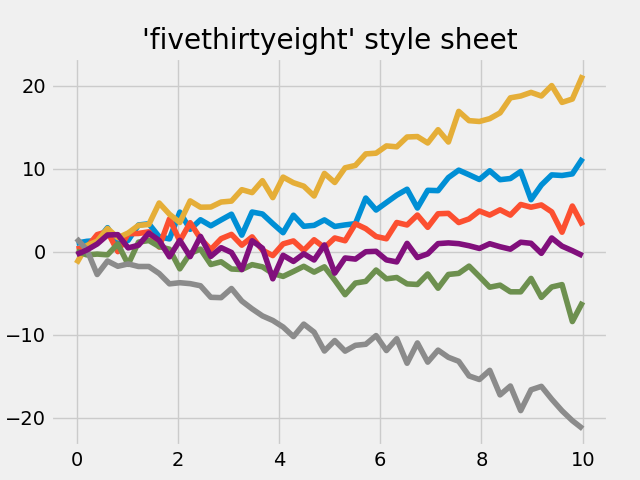Travis-CI:# FiveThirtyEight style sheet¶

This shows an example of the “fivethirtyeight” styling, which tries to replicate the styles from FiveThirtyEight.com.```from matplotlib import pyplot as plt
import numpy as np

plt.style.use('fivethirtyeight')

x = np.linspace(0, 10)

# Fixing random state for reproducibility
np.random.seed(19680801)

fig, ax = plt.subplots()

ax.plot(x, np.sin(x) + x + np.random.randn(50))
ax.plot(x, np.sin(x) + 0.5 * x + np.random.randn(50))
ax.plot(x, np.sin(x) + 2 * x + np.random.randn(50))
ax.plot(x, np.sin(x) - 0.5 * x + np.random.randn(50))
ax.plot(x, np.sin(x) - 2 * x + np.random.randn(50))
ax.plot(x, np.sin(x) + np.random.randn(50))
ax.set_title("'fivethirtyeight' style sheet")

plt.show()
```

Total running time of the script: ( 0 minutes 0.021 seconds)

Gallery generated by Sphinx-Gallery